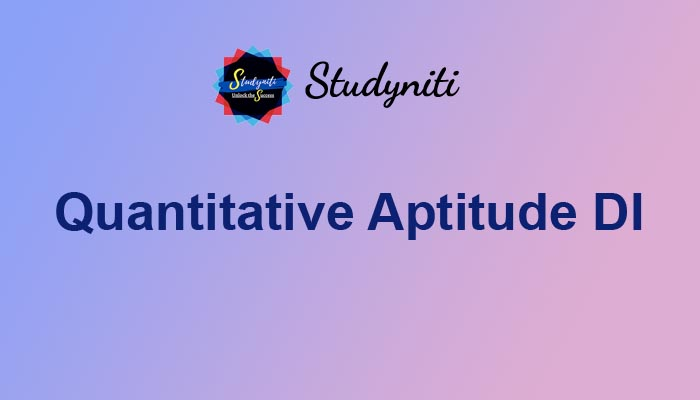## Payment Processing...# Quantitative Aptitude DI - Nov 07 2020

Here we are providing new series of Quantitative Aptitude Questions for upcoming exams, so the aspirants can practice it on a daily basis.

Study the following information carefully and answer the questions given below.

The bar chart given below shows the percentage of quantity of wine, soda and water in tank A, tank B, tank C and tank D and line chart given below shown the capacity of the tank A, tank B, tank C and tank D. Study the data carefully and answer the following questions. (Note- all the tanks are filled to its full capacity)1) If 20% of the quantity of tank A and 30% of the quantity of tank B are mixed in tank E. Find the amount of water in tank E.

A.34.4 litres

B.32.4 litres

C.31.4 litres

D.31.6 litres

E.30.4 litres

2) If any three tanks are mixed in a large tank then the maximum quantity of wine can be obtained from which combination?

A. Tank A + Tank B + Tank C

B. Tank A + Tank B + Tank D

C. Tank A + Tank C + Tank D

D. Tank B + Tank C + Tank D

E. Both option c and d are correct.

3) Find the ratio of the quantity of wine, soda and water respectively in a glass if the 40ml liquid is taken from tank C and 60 ml liquid is tank from tank D.

A.33: 37: 30

B.30: 37: 33

C.37: 33: 30

D.40: 33: 27

E.33: 47: 20

4) If the price of wine is Rs. 600/litre and price of soda is Rs. 80/litre. Find the amount of profit obtained by selling the whole mixture of tank C at the rate of Rs. 500/litre.

A.Rs. 44000

B.Rs. 54000

C.Rs. 34000

D.Rs. 36000

E.Rs. 64000

5) The total quantity of soda in all the tanks is approximately what percent of the total quantity of wine in all the tanks?

A.110%

B.125%

C.120%

D.130%

E.135%

Answers :

1) Answer: E

Tank A

20% of 160 = 32 litres

Quantity of water = 50% of 32 = 16 litres

Tank B

30% of 120 = 36 litres

Quantity of water = 40% of 36 = 14.4 litres

Quantity of water in tank E = 16 litres + 14.4 litres = 30.4 litres

2) Answer: C

Quantity of wine

Option a) = 20% of 160 + 25% of 120 + 30% of 180

= 32+ 30+ 54

=116 litres

Option b) 20% of 160 + 25% of 120 + 35% of 150

= 32+30 + 52.5

= 114.5 litres

Option c) 20% of 160+ 30% of 180+ 35% of 150

= 32+ 54+ 52.5

= 138.5 litres (answer)

Option d) = 25% of 120 + 30% of 180+35% of 150

= 30+ 54+ 52.5

= 136.5 litres

3) Answer: A

Tank C

=wine (30% of 40ml) + soda (25% of 40 ml) + water (45% of 40ml)

40ml = wine (12ml) + soda (10ml) + water (18ml)

Tank D

= wine (35% of 60ml) + soda (45% of 60ml) + water (20% of 60ml)

60ml = wine (21ml) + soda (27 ml) + water (12ml)

Glass

Wine (12ml+21ml) + soda (10ml+27ml) + water (18ml+12ml)

Wine (33ml) + soda (37ml) + water (30ml)

Required ratio = 33: 37: 30

4) Answer: B

Selling price of tank C liquid = 180 * 500 = Rs. 90000

Cost price of tank C liquid = cost of wine + cost of soda

Cost price of tank C liquid = quantity of wine * 600 + quantity of soda * 80

Cost price of tank C liquid = (30% of 180) * 600 + (25% of 180) * 80

Cost price of tank C liquid = 54 * 600 + 45 * 80

Cost price of tank C liquid = 32400 + 3600

Cost price of tank C liquid = 36000

Required profit = 90000 - 36000 = Rs. 54000

5) Answer: C

Quantity of soda = 30% of 160 + 35% of 120 + 25% of 180 + 45% of 150

= 48+ 42+45+ 67.5

= 202.5

Quantity of wine = 20% of 160 + 25% of 120 + 30% of 180+ 35% of 150

= 32 + 30 + 54 + 52.5

= 168.5

Required %age = (202.5/168.5)*100 = 120%